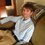# Inverse Functions and their intersection points

Say you have $f(x)$ and $g(x)$ and $g(x) = f^{-1}(x)$.

I observed that these two curves need not intersect, for example with $f(x) = e^x$ and $g(x) = \ln x$ never intersecting each other.

I also observed that a function can either have one, two, or three intersections with its inverse, but I was unable to find a function which has more than 3 intersection points with its inverse.

How would I prove or disprove the hypothesis that an elementary function and its intersection can only have up to 3 intersection points? Any counterexamples are appreciated!Note by Oli Hohman
5 years ago

This discussion board is a place to discuss our Daily Challenges and the math and science related to those challenges. Explanations are more than just a solution — they should explain the steps and thinking strategies that you used to obtain the solution. Comments should further the discussion of math and science.

When posting on Brilliant:

• Use the emojis to react to an explanation, whether you're congratulating a job well done , or just really confused .
• Ask specific questions about the challenge or the steps in somebody's explanation. Well-posed questions can add a lot to the discussion, but posting "I don't understand!" doesn't help anyone.
• Try to contribute something new to the discussion, whether it is an extension, generalization or other idea related to the challenge.

MarkdownAppears as
*italics* or _italics_ italics
**bold** or __bold__ bold
- bulleted- list
• bulleted
• list
1. numbered2. list
1. numbered
2. list
Note: you must add a full line of space before and after lists for them to show up correctly
paragraph 1paragraph 2

paragraph 1

paragraph 2

[example link](https://brilliant.org)example link
> This is a quote
This is a quote
    # I indented these lines
# 4 spaces, and now they show
# up as a code block.

print "hello world"
# I indented these lines
# 4 spaces, and now they show
# up as a code block.

print "hello world"
MathAppears as
Remember to wrap math in $$ ... $$ or $ ... $ to ensure proper formatting.
2 \times 3 $2 \times 3$
2^{34} $2^{34}$
a_{i-1} $a_{i-1}$
\frac{2}{3} $\frac{2}{3}$
\sqrt{2} $\sqrt{2}$
\sum_{i=1}^3 $\sum_{i=1}^3$
\sin \theta $\sin \theta$
\boxed{123} $\boxed{123}$

Sort by:

Consider $f(x) = x$.

Note that $g(x) = f^{-1}(x) = x = f(x)$.

So, there are infinitely many intersections!

Good solution. I am aware of the infinite intersections solution, but does anyone have any functions which have 4 or more intersections with their inverse (but not an infinite number of intersections)?

- 5 years ago

Consider, over any finite interval say $X$, $f(x) = x + \sin x$.

Over the interval $X$ there are finitely many intersections. The exact number depends on $X$ itself. But you can have any finite number of intersections.

Nice solution

- 4 years, 12 months ago

At first, I was unsure of how to find the inverse of that function, so I decided to graph it to verify your claim and you're right!

http://www.wolframalpha.com/input/?i=find+inverse+of+f(x)+%3D+x%2Bsin(x)

- 4 years, 8 months ago